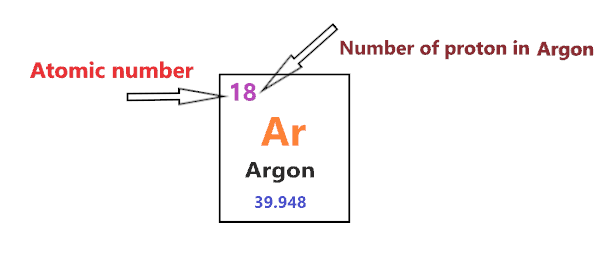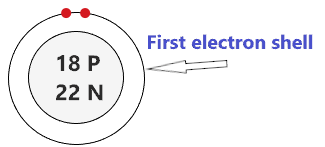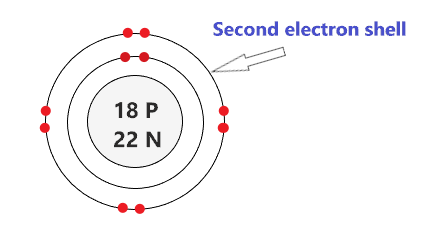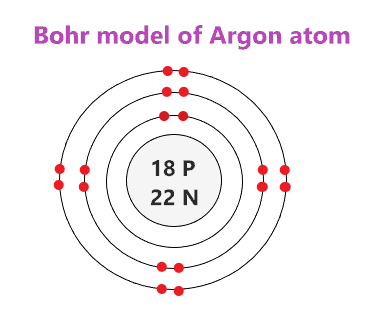# Bohr model of Argon atom - How to draw Argon(Ar) Bohr-Rutherford diagram?

Home  > Chemistry Article > Argon Bohr model

The Bohr Model of Argon(Ar) has a nucleus that contains 22 neutrons and 18 protons. This nucleus is surrounded by three-electron shells named K-shell, L-shell, and M-shell. The outermost shell in the Bohr diagram of Argon contains 8 electrons that also called valence electrons.

 Name Argon Bohr Model Number of neutrons 22 Number of protons 18 Number of electrons 18 Total electron shells 3 Electron in the First shell(K) 2 Electrons in the Second shell(L) 8 Electrons in the Third shell(M) 8 Total valence electrons in Argon 8
Page Contents

## How to draw Bohr Model of Argon(Ar)?

Bohr model describes the visual representation of orbiting electrons around the small nucleus. It used different electron shells such as K, L, M, N…so on. These shells hold a specific number of electrons, the electron shell which is closest to the nucleus has less energy and the electron shell which is farthest from the nucleus has more energy.

Bohr diagram is very interesting and easy to draw. Here, we will draw the Bohr diagram of the Argon atom with some simple steps.

## Steps to draw the Bohr Model of Argon atom

1. Find the number of protons, electrons, and neutrons in the Argon atom

Protons are the positively charged particles and neutrons are the uncharged particles, both these are constituents of the atom nuclei. Electrons are the negatively charged particles that orbit the nucleus of an atom

To find the number of protons an atom contains, just look at their atomic number.

If the atomic number of atom is 31, then proton will also be 31, if atomic number is 33, then proton will also be 33.

So, the atomic number for Argon is 18, hence, the number of protons in the Argon atom is also 18.Now, to determine the number of neutrons in an atom, use this formula.

⇒ Number of neutrons in atom = Atomic mass of the atom(rounded to the nearest whole number) – Number of proton in an atom

For example, An atom have 33.977 atomic mass and 20 protons.

Then, to find the number of neutron, round the atomic mass to the near whole number, so, atomic mass 33.977 round to 34.

= (34 – 20 protons) = 14 number of neutrons in the atom

Now, To get the number of neutrons in an Argon atom, look at its atomic mass which is 39.948 rounded to 40, and the number of protons in Argon is 18.

∴ Hence, the number of neutrons in Argon atom = (40 – 18) = 22It should be noted that “The number of electrons in a neutral atom is equal to the number of protons”.

So, the Argon atom is neutral, hence, its number of electrons will be equal to the number of protons which is 18 as we already discussed.

⇒ The number of electrons in Argon atom = 18

⇒ The number of protons in Argon atom = 18

⇒ The number of neutrons in Argon atom = 22

2. Draw the nucleus of an atom

A nucleus is a dense and small region that contains the number of protons and neutrons of an atom.

In this step, we have to draw a small circle that consists of a number of protons and the number of neutrons of an Argon atom.3. Draw the First electron shell

“An electron shell may be thought of as an orbit followed by electrons around an atom’s nucleus.”

The first electron shell is also called the K-shell, this is the closest shell to the nucleus of an atom and can hold a maximum of two electrons.

As we know, the Argon atom has a total of 18 electrons. So, put two electrons from it, in the first shell, next to each other.We have successfully drawn the first shell of the Argon atom that can hold 2 electrons. As an Argon atom has a total of 18 electrons, and from 18 electrons we have used two electrons in the first shell.

∴ (18 – 2) = 16 electrons

Therefore, we are left with 16 electrons, let’s put them in the next shells of the Argon atom.

4. Draw the Second electron shell

The second shell also called the L-shell that can hold a maximum of 8 electrons. This shell is drawn after the first electron shell.

In the second electron shell, the electrons are added one at a time, starting from the top position then going in a clockwise direction.

In second shell, electrons are added one at a time in clockwise direction as a clock position – 12 o’clock, 3 o’clock, 6 o’clock, 9 o’clock positions.Once you place the electrons one at a time to each of the four sides(Top – Right – Bottom – Left], start pairing or doubling them.

So, we have 16 remaining electrons of an Argon atom, and the 2nd shell can only hold a maximum of 8 electrons.

Therefore, put the 8 electrons of the Argon atom in the 2nd electron shell, start from the top position, put electrons one at a time, and go in a clockwise direction(Top – Right – Bottom – Left). And, finally, pair them up.As we have a total of 18 electrons for the Argon atom and we placed 2 electrons in the first shell and 8 electrons in the second shell, hence, we are left with 8 electrons more.

Let’s place it in the next shell.

5. Draw the Third electron shell

The third shell is also called the M-shell and it has the capacity to hold up to 18 electrons but, for the elements in the first few periods, the third shell only holds up to 8 electrons.

“The third shell can be considered to hold 8 or 18 electrons but in total the third shell can hold 18 electrons.”

For the first few elements, say, till atomic number 20 on the periodic table, the third shell holds up to 8 electrons.

⇒ For atomic number 20 or less than 20, the third shell can hold up to maximum of 8 electrons.

⇒ For the atomic number more than 20, say, atomic numbers 21, 22, 23, 24, 25, 26, 27,…….etc., the third shell is filled with more than 8 electrons and up to maximum of 18 electrons.

For the Argon atom, the third shell is filled with the same procedure as the second shell.

So, we have left with 8 electrons of an Argon atom, so, start from the top position, put electrons one at a time, and go in a clockwise direction(Top – Right – Bottom – Left). And, finally, start pairing.### Bohr model of Argon atom

That’s all, this is our Bohr model of the Argon atom that contains 18 protons and 22 neutrons in the nucleus region, and 18 electrons are orbited around the nucleus, two electrons in the first shell, eight electrons in the second shell, and eight electrons in the third shell.

## Find Valence electron of Argon through its Bohr diagram

Valence electrons are found in the outermost shell of an atom and they can take participate in the formation of a chemical bond. These electrons have more energy compare to the inner shell electrons.

From the Bohr diagram of an atom, we can easily find the number of valence electrons in an atom by looking at its outermost shell.

So, we have to find a valence electron in the Argon atom, for this, look at its Bohr diagram.

Bohr’s diagram of Argon has three electron shells (K, L, and M), the inner shell is K-shell and the outermost shell is M-shell.

Hence, the electrons found in the M-shell of the Argon atom are its valence electrons because it is the outermost shell that also called the valence shell.

The M-shell or outer shell of the Argon Bohr model contains 8 electrons, therefore, the number of the valence electrons in the Argon atom is also 8.## Electron dot diagram of an Argon atom

Electron dot diagram also called lewis structure which represents the valence electrons of atoms.

As, from the Bohr diagram of Argon, we got to know, it has 8 valence electrons.

So, just represent the 8 valence electrons around the Argon atom as a dot.## The electron configuration of Argon

Electron configuration is the distribution of electrons of an atom or molecule in atomic or molecule orbitals”

Argon has an atomic number of 18 and it contains a total number of 18 electrons. From the Bohr model of Argon, we know, 2 electrons are in the K-shell, 8 electrons are in the L-shell, and 8 electrons are in the M-shell.

So, based on the shell, the electronic configuration of the Argon atom is [2, 8, 8].

Or the electronic configuration of Argon is 1s22s22p63s23p6 since it contains a total of 18 electrons.## FAQ

### How many electron shells an Argon Bohr model contains?

Electron shell also called energy level, you can find the number of electron shells for an element by knowing its period number in the periodic table.

The elements or atoms in the first period of the periodic table have one energy level or one electron shell, same as, the elements in the second period have two energy levels or two-electron shells and so on…

So, the Argon atom belongs to the 3rd Period in the periodic table, hence, the number of electron shells for the Bohr model of Argon will be 3 (K-shell, L-shell, and M-shell).

### What is the outer shell of the Bohr diagram of the Argon atom?

The outermost shell also called the valence shell, which contains valence electrons of the atom.

According to the Bohr diagram of Argon, the outer shell is M-shell which contains eight valence electrons.

## Properties of Argon

1. It is the third-most abundant gas in the Earth’s atmosphere.
2. It exhibits a violet glow when placed in an electric field.
3. It has a boiling point of −185.848 °C and a melting point of −189.34 °C.
4. It is 2.5 times more soluble in water than nitrogen.
5. It has a face-centered cubic crystal structure.

## Summary

• The Bohr model of Argon(Ar) is drawn with three electron shells, the first shell contains 2 electrons, the second shell contains 8 electrons and the third shell contains 8 electrons.
• Argon is neutral and its atomic number is 18, hence, the number of protons and electrons available for its Bohr diagram is also 18.
• The number of neutrons for the Bohr diagram of Argon can be found by subtracting the number of protons from the atomic mass(rounded to the nearest whole).
• The electron configuration of Argon in terms of the shell is [2, 8, 8], or in normal form, it is [Ne] 3s23p6.
##### Subscribe to Blog via Email

Join 2 other subscribers

Share it...